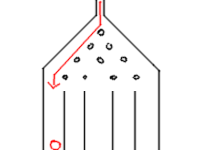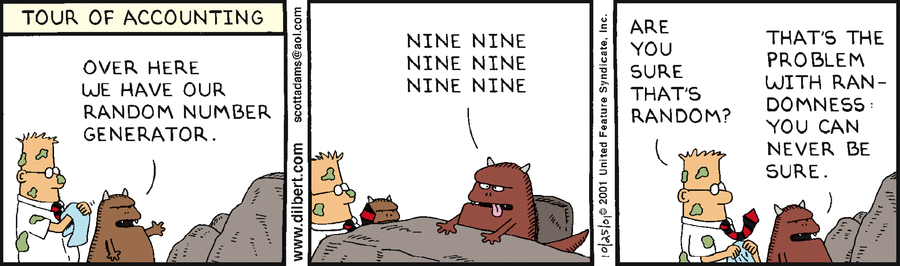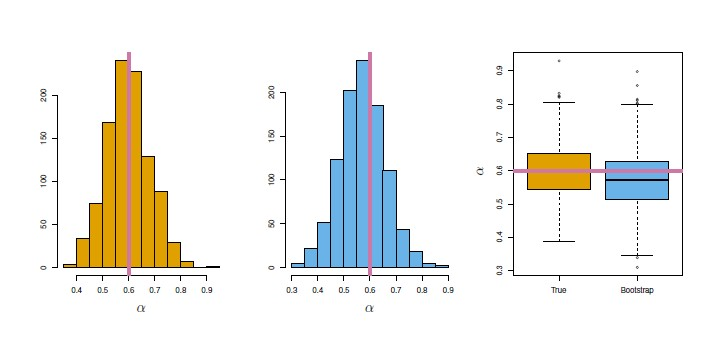# Statistics - Central limit theorem (CLT)

The central limit theorem (CLT) is a probability theorem (unofficial sovereign)

It establishes that when:

The first version of this theorem was postulated by the French-born mathematician Abraham de Moivre who, in a remarkable article published in 1733, used the normal distribution to approximate the distribution of the number of heads resulting from many tosses of a fair coin.

The actual term “central limit theorem” (in German: “zentraler Grenzwertsatz”) was first used by George Pólya in On the central limit theorem of calculus of probability and the problem of moments (German). He uses the term central to emphasize its importance in probability theory.

## More

The sum of k random variables (independent) approaches a normal distribution as k increases,

The central limit theorem began in 1733 when de Moivre approximated binomial probabilities using the integral of $exp(-x^2)$ (gaussian_function) The central limit theorem achieved its final form around 1935 in papers by Feller, Lévy, and Cramér.

The central limit theorem is a fundamental component of inferential statistics

The theorem is a key concept in probability theory because it implies that probabilistic and statistical methods that work for normal distributions can be applicable to many problems involving other types of distributions.

## Application

### Random Samples

The central limit theorem says that the averages of several samples obtained from the same population (ie a sampling distribution) following the central limit theorem rules (see below) will be distributed according to the normal distribution.

Therefore:

The population doesn't have to be normally distributed, as long as we get multiple samples of large enough size (N>30) then the sampling distribution will take on a normal distribution.

Rules

• The sample must contain a large number of observations (N>30)
• Each observation must be randomly generated (No relationship/dependencies between the observations)
• The shape of the distribution of sample means is always normal (not negatively or positively skewed, not uniform)

Creation of a sampling distribution based on the mean estimator.

• Creating the population data randomly distributed
population_n = 10000;
population_data = [];
population_max = 100;
population_data = [];

for (i = 0; i < population_n; i++) {
random_value = Math.floor(Math.random() * Math.floor(population_max));
population_data.push(random_value);
}

histogram({ selector: "population", data: population_data});

• Sampling the population 1000 times with a sample size of 20, calculating the mean and adding it to the sample distribution
// Sample Data
sample_distribution_data = [];
sample_distribution_n = 1000;
for (j = 0; j < sample_distribution_n; j++) {
sample_data = [];
sample_n = 20;
for (i = 0; i < sample_n; i++) {
population_random_index = Math.floor(
Math.random() * Math.floor(population_max)
);
sample_data.push(population_data[population_random_index]);
}
sample_distribution_data.push(d3.mean(sample_data));
}
histogram({ selector: "sample", data:sample_distribution_data});


### Tosses of a fair coin

Coin Probability of getting a given number of heads in a series

If you flipped a coin 10 times over and over. You would expect to get 5 heads and 5 tails most often, but would get 6 and 4 sometimes, and so on, with normal distribution.

A simple example of this is that if one flips a coin many times the probability of getting a given number of heads in a series of flips will approach a normal curve, with mean equal to half the total number of flips in each series. In the limit of an infinite number of flips, it will equal a normal curve.

• Creating the coin flip simulation
flip_n = 50;
for (i = 0; i < head_distribution_n; i++) {
flip_results = [];
for (j = 0; j < flip_n ; j++){
flip_value = Math.round(Math.random()); // 0 or 1
flip_results.push(flip_value );
}
}

histogram({
bins: flip_n,
min: 0,
max: flip_n
});


### Errors of measurements

The occurrence of the Gaussian probability density in errors of measurements, which result in the combination of very many and very small elementary errors, in diffusion processes etc., can be explained, by the very same limit theorem.

The central limit theorem explains the common appearance of the “bell curve” in density estimates applied to real world data. In cases like electronic noise, examination grades, and so on, we can often regard a single measured value as the weighted average of many small effects.

Demo with the error of a pseudo-random number generator:

• Creating the population data (10000) randomly generated with value between 0 and 100
population_n = 10000;
population_data = [];
population_max = 100;
population_data = [];

for (i = 0; i < population_n; i++) {
random_value = Math.floor(Math.random() * Math.floor(population_max));
population_data.push(random_value);
}

• Calculating the errors for each bin
// The length of each bins
lengths = bins.map(function (d) { return d.length })
// The mean of the length of each bean
lengths_mean = d3.mean(lengths)
console.log("Mean of each length bin = "+lengths_mean)
// The errors (mean - length of each bin)
errors = bins
.filter(function(d) { return Math.abs(d.length - lengths_mean) < 50 }) // One outlier, why ?
.map(function(d) { return d.length - lengths_mean; } )

// Plotting the errors
errors_min = d3.min(errors)
errors_max = d3.max(errors)
errors_bins = d3.histogram()
.domain([errors_min,errors_max]) // then the domain of the graphic
.thresholds(30)
(errors ); // 30 bins
histogram_graphic({ selector: "error", data: errors , bins: errors_bins });


### Galtonboard

The Galton board is a physical model of the binomial distribution which beautifully illustrates the central limit theorem.

It is a visual proof (not a rigorous one) of the central limit theorem where:

• The variable is whether it goes left or right. (The ball is not the variable.)
• The randomness comes from the fact that every ball is randomly pushed left and right. The choice at each peg remains binary and random (50/50).
• The final random variable is the bin

More … see Galton board

## Documentation / Reference

Discover MoreGalton board

The is a physical model of the binomial distribution which beautifully illustrates the central limit theorem Galtonboard is also known as: Galtonbrett Simulation, quincunx bean machine or...Number - Random (Stochastic|Independent) or (Balanced)

Think of randomness as a lack of pattern. Something random should be unpredictable. We shouldn’t be able to predict the next value of the sequence The degree to which a system has no pattern is known...Statistics - (Normal|Gaussian) Distribution - Bell Curve

A normal distribution is one of underlying assumptions of a lot of statistical procedures. In nature, every outcome that depends on the sum of many independent events will approximate the Gaussian distribution...Statistics - (Student's) t-test (Mean Comparison)

The t-test is a test that compares means. NHST can be conducted yielding to a p-value Effect Size can be calculated like in multiple regression. Confidence Interval around the mean can also be...Statistics - Bootstrap Resampling

Bootstrap is a powerful resampling method for assessing uncertainty in estimates and is particularly good for getting their: standard errors and confidence limits. Why is the bootstrap useful? The...Statistics - Random Variable (Random quantity|Aleatory variable|Stochastic variable)

Random variable is also known as: random quantity, aleatory variable, or stochastic variable A random variable represents the result of a random process. The random variable value is the summary...Statistics - Sampling Distribution

Distribution of estimated statistics from different samples (same size) from the same population is called a sampling distribution sampling distributionsample distribution It permits to make probability...Statistics / Probability - Gaussian function ( )

In statistics and probability theory, Gaussian functions appear as the density function of the normal distribution, which is a limiting probability distribution of complicated sums, according to the central...